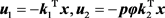# 时滞广义变结构控制研究新进展New Research Advance of Variable Structure Control Singular Systems with Time Delays

DOI: 10.12677/DSC.2018.74038, PDF, HTML, XML, 下载: 520  浏览: 1,242  科研立项经费支持

Abstract: Firstly, seven methods are discussed exhaustively for the variable structure control singular sys-tems with time-delays, including the method of sliding mode approximation, the method of soft variable structure control, restricted synthesis method, the method of adaptive sliding mode con-trol and others. Secondly, so far some research advances in this system are summarized. Finally, some new research directions for relevant variable structure control singular systems with time delays are pointed out.

1. 引言

$\left\{\begin{array}{c}E\left(x,t\right)\stackrel{˙}{x}=f\left(x,u,t\right)\\ y=g\left(x,u,t\right)\end{array}$ (1)

2. 变结构控制与广义系统概述

2.1. 变结构概述

2.2. 广义系统概述

3. 时滞广义变结构控制系统研究方法

3.1. 滑模近似法

$\left\{\begin{array}{l}E\stackrel{˙}{x}\left(t\right)=\left(A+\text{Δ}A\right)x\left(t\right)+\left(B+\text{Δ}B\right)x\left(t-\tau \right)+\left(F+\text{Δ}F\right)u\left(t\right)+Dg\left(t\right)\\ x\left(t\right)=\phi \left(t\right),t\in \left[-\tau ,0\right]\end{array}$ (2)

3.2. 受限综合法

$\left\{\begin{array}{l}E\stackrel{˙}{x}\left(t\right)=A\left(t\right)x\left(t\right)+f\left(t,x\left(t\right),x\left(t-\tau \right)\right)+B\left(t\right)u\left(t\right)\\ x\left(t\right)=\phi \left(t\right),t\in \left[-\tau ,0\right]\end{array}$ (3)

1) 若 $rank\left(B\left(t\right),f\right)=rank\left(B\left(t\right)\right)$ ，则系统(3)受限等价于：

$\left\{\begin{array}{l}\stackrel{˙}{x}\left(t\right)={A}_{\text{11}}\left(t\right){x}_{\text{1}}\left(t\right)+{A}_{\text{12}}\left(t\right){x}_{\text{2}}\left(t\right)\\ \text{0}={A}_{\text{21}}\left(t\right){x}_{\text{1}}\left(t\right)+{A}_{\text{12}}\left(t\right){x}_{\text{2}}\left(t\right)\\ \text{}+{f}_{\text{2}}\left(t,{x}_{\text{1}}\left(t\right),{x}_{\text{2}}\left(t\right),{x}_{\text{1}}\left(t-\tau \right),{x}_{\text{2}}\left(t-\tau \right)\right)+{B}_{\text{2}}\left(t\right)u\left(t\right)\\ \left[\begin{array}{c}{x}_{\text{1}}\left(t\right)\\ {x}_{\text{2}}\left(t\right)\end{array}\right]={P}^{-\text{1}}\phi \left(t\right)=\left[\begin{array}{c}{\phi }_{\text{1}}\left(t\right)\\ {\phi }_{2}\left(t\right)\end{array}\right],t\in \left[-\tau ,0\right]\end{array}$ (4)

2) 若 $rank\left(B\left(t\right),f\right)\ne rank\left(B\left(t\right)\right)$ ，则系统(3)受限等价于：

 (5)

3.3. 软变结构控制法

$\stackrel{˙}{x}\left(t\right)=Ax\left(t\right)+bu\left(t\right)$ (6)

$|u\left(t\right)|\le {u}_{0}$ (7)

$u\left(t\right)=-{k}_{p}^{T}x\left(t\right),p=1,2,\cdots ,k$ (8)

2) 动态软变结构控制：假设所选择的策略参量 $p$ 满足 $\stackrel{˙}{p}=f\left(p,x\right)$ ，则设计的控制器为：

$u=-{g}^{T}x-p{h}^{T}x$ (9)

$\stackrel{˙}{x}=\left(A-b{g}^{T}\right)x-pb{h}^{T}x=\left({A}_{0}-pb{h}^{T}\right)x$ (10)

3) S-型函数软变结构控制：构造两个向量 ${k}_{\text{1}}\in {R}^{n},{k}_{\text{2}}\in {R}^{n}$ ，使得(11)

$u={u}_{\text{1}}+{u}_{\text{2}}$ (12)

$\stackrel{˙}{x}=\left(A-b{k}_{1}{}^{T}-\rho \phi b{k}_{2}{}^{T}\right)x\underset{_}{\underset{_}{\Delta }}\stackrel{¯}{A}\left(\rho \right)x$ (13)

3.4. 趋近律法

3.4.1. 趋近律的研究状况

$\begin{array}{c}{s}_{2}\left(k+2\right)=f\left[{s}_{2}\left(k+1\right)\right]f\left[{s}_{2}\left(k\right)\right]{s}_{2}\left(k\right)-\alpha \mathrm{sgn}\left[{s}_{2}\left(k+1\right)\right]-f\left[{s}_{2}\left(k+1\right)\right]\alpha \mathrm{sgn}\left[{s}_{2}\left(k\right)\right]\\ +{c}_{2}{}^{\text{T}}Ab\stackrel{¯}{\alpha }\left(k\right)+{c}_{2}{}^{\text{T}}Abd\left(k\right)-{d}_{2}\end{array}$ (14)

$\stackrel{˙}{S}=-\frac{k{|s|}^{\alpha }}{N|s|}sign\left(S\right)$ (15)

3.4.2. 理想趋近律与扰动补偿趋近律

3) 稳定于切换面时速度趋于零，即 $\underset{k\to \infty }{\mathrm{lim}}s\left(k+1\right)=s\left(k\right)$

$s\left(k+1\right)=\left(1-qT\right)s\left(k\right)-\epsilon Tsgns\left(k\right)+{c}^{\text{T}}g\left(k\right)-\rho sgns\left(k\right)-\underset{i=2}{\overset{k}{\sum }}\left[s\left(i\right)-I\left(\alpha \right)s\left(i-1\right)+\rho sgns\left(i-1\right)\right]$ (16)

3.5. 受限等价法

1) 非结构式扰动的单时滞情况；2) 具有结构式扰动的单时滞情况；3) 非结构式扰动的多时滞情况；4) 具有结构式扰动的多时滞情况。

3.6. 自适应滑模控制法

$u\left(k\right)=-{\left(CB\right)}^{-1}\left[CBKx\left(k\right)+Ksign\left(s\left(k\right)\right)+sign\left(s\left(k\right)\right){A}_{x}\right]$ (17)

$sign\left(s\left(k\right)\right)=\left[\begin{array}{c}sign\left({s}_{1}\left(k\right)\right)\\ ⋮\\ sign\left({s}_{m}\left(k\right)\right)\end{array}\right]$$K=\left[\begin{array}{ccc}{K}_{1}& 0& 0\\ 0& \ddots & 0\\ 0& 0& {K}_{m}\end{array}\right]$

Gracia  等研究了一类机器人自适应滑模控制问题，通过考虑和比较几种自适应开关增益，来分析所产生的不稳定性及其其他性能；Liu  等研究的是一类离散非线性系统的无记忆自适应滑模控制，设计的控制器如下所示：

${u}_{k}={u}_{k-1}+{\Phi }_{2,k}^{-1}\left({e}_{k}-\Delta {y}_{k}{\Phi }_{1,k}-\frac{{\stackrel{¯}{\epsilon }}_{k+1}\left(\eta {\theta }_{k}+1-\eta \right)-{\underset{_}{\epsilon }}_{k+1}}{\eta {\theta }_{k}+2-\eta }{\vartheta }_{k+1}\right)+{\Phi }_{2,k}^{-1}{\Lambda }_{s}\text{sign}\left({s}_{k}\right)$ (18)

$u={\phi }^{-1}\left[{\stackrel{¨}{q}}_{d}+\alpha {\stackrel{˙}{e}}^{\frac{q}{p}}+\beta {e}^{\frac{q}{2p-q}}-f+\lambda s+{\phi }^{T}\stackrel{^}{A}\frac{s}{‖s‖}\right]$ (19)

Zhang  等是采用鲁棒自适应积分滑模控制方法，提出了一种基于动态滞后补偿的控制方法，研究了一类具有时间滞后和不确定性的系统的稳定性问题，设计了一种自适应律来估计积分滑模控制器的增益，从而削弱了系统抖振，且不再需要先验的不确定性上界有界的约束条件。采用自适应滑模控制法来设计控制器，不仅能够保证系统的鲁棒性，而且能够对系统的不确定性和外界干扰性进行补偿。

3.7. 随机广义控制法

4. 几个新的研究方向

1) 模糊时滞广义VSC系统的分析与综合；2) 冗余时滞广义VSC系统的分析与综合；3) 不确定时滞广义VSC系统的分析与综合；4) 随机时滞广义VSC系统的分析与综合；5) 分布参数时滞广义VSC系统的分析与综合；6) 时滞广义VSC系统的定向分析综合与应用；7) 时滞广义VSC系统的智能控制理论与应用；8) 时滞广义VSC系统在各类实际中的应用分析；9) 基于神经网络的时滞广义VSC系统的应用研究；10) 分数阶时滞广义VSC系统的分析与综合；11) 耦合时滞广义VSC系统的分析与综合；12) 混沌时滞广义VSC系统的分析与综合等。

5. 结语

NOTES

*通讯作者。